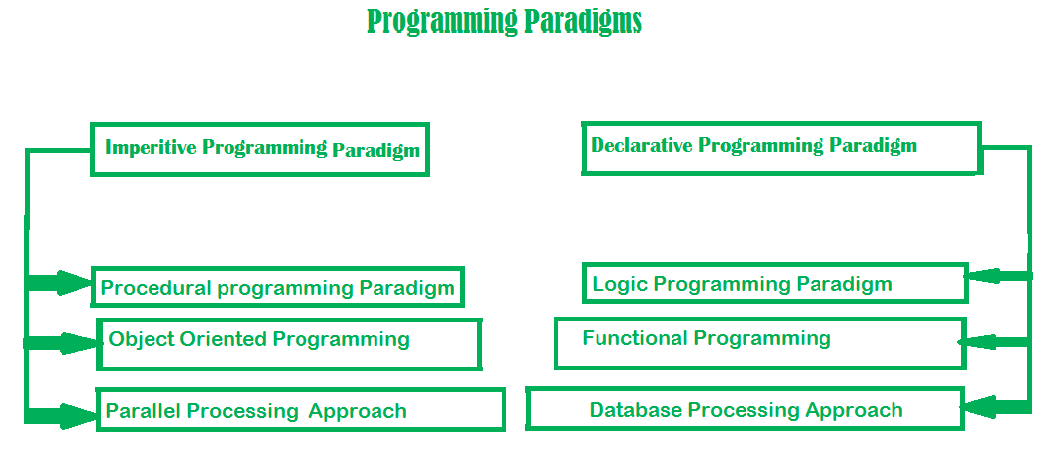Paradigm can also be termed as a method to solve some problems or do some tasks. A programming paradigm is an approach to solve the problem using some programming language or also we can say it is a method to solve a problem using tools and techniques that are available to us following some approach. There are lots of programming languages that are known but all of them need to follow some strategy when they are implemented and this methodology/strategy is paradigms. Apart from varieties of programming languages, there are lots of paradigms to fulfill each and every demand.Python supports three types of Programming paradigms

In the object-oriented programming paradigm, objects are the key element of paradigms. Objects can simply be defined as the instance of a class that contains both data members and the method functions. Moreover, the object-oriented style relates data members and methods functions that support encapsulation and with the help of the concept of an inheritance, the code can be easily reusable but the major disadvantage of object-oriented programming paradigm is that if the code is not written properly then the program becomes a monster.

• Relation with Real world entities
• Code reusability
• Abstraction or data hiding

• Data protection
• Not suitable for all types of problems
• Slow Speed

Example:

 `# class Emp has been defined here ` `class` `Emp: ` `    ``def` `__init__(``self``, name, age): ` `        ``self``.name ``=` `name ` `        ``self``.age ``=` `age ` `     `  `    ``def` `info(``self``): ` `        ``print``(``"Hello, % s. You are % s old."` `%` `(``self``.name, ``self``.age)) ` ` `  `# Objects of class Emp has been  ` `# made here         ` `Emps ``=` `[Emp(``"John"``, ``43``), ` `    ``Emp(``"Hilbert"``, ``16``), ` `    ``Emp(``"Alice"``, ``30``)] ` ` `  `# Objects of class Emp has been ` `# used here ` `for` `emp ``in` `Emps: ` `    ``emp.info() `

Output:

```Hello, John. You are 43 old.
Hello, Hilbert. You are 16 old.
Hello, Alice. You are 30 old.```

In Procedure Oriented programming paradigms, series of computational steps are divided modules which means that the code is grouped in functions and the code is serially executed step by step so basically, it combines the serial code to instruct a computer with each step to perform a certain task. This paradigm helps in the modularity of code and modularization is usually done by the functional implementation. This programming paradigm helps in an easy organization related items without difficulty and so each file acts as a container.

• General-purpose programming
• Code reusability
• Portable source code

• Data protection
• Not suitable for real-world objects
• Harder to write

Example:

 `# Procedural way of finding sum  ` `# of a list  ` ` `  `mylist ``=` `[``10``, ``20``, ``30``, ``40``] ` ` `  `# modularization is done by  ` `# functional approach ` `def` `sum_the_list(mylist): ` `    ``res ``=` `0` `    ``for` `val ``in` `mylist: ` `        ``res ``+``=` `val ` `    ``return` `res ` ` `  `print``(sum_the_list(mylist)) `

Output:

`100`

Functional programming paradigms is a paradigm in which everything is bind in pure mathematical functions style. It is known as declarative paradigms because it uses declarations overstatements. It uses the mathematical function and treats every statement as functional expression as an expression is executed to produce a value. Lambda functions or Recursion are basic approaches used for its implementation. The paradigms mainly focus on “what to solve” rather than “how to solve”. The ability to treat functions as values and pass them as an argument make the code more readable and understandable.

• Simple to understand
• Making debugging and testing easier
• Enhances the comprehension and readability of the code

• Low performance
• Writing programs is a daunting task
• Low readability of the code

Example:

 `# Functional way of finding sum of a list  ` `import` `functools ` ` `  ` `  `mylist ``=` `[``11``, ``22``, ``33``, ``44``] ` ` `  `# Recursive Functional approach ` `def` `sum_the_list(mylist): ` `     `  `    ``if` `len``(mylist) ``=``=` `1``: ` `        ``return` `mylist[``0``] ` `    ``else``: ` `        ``return` `mylist[``0``] ``+` `sum_the_list(mylist[``1``:]) ` ` `  `# lambda function is used ` `print``(functools.``reduce``(``lambda` `x, y: x ``+` `y, mylist)) `

Output:

`110`

Attention geek! Strengthen your foundations with the Python Programming Foundation Course and learn the basics.

To begin with, your interview preparations Enhance your Data Structures concepts with the Python DS Course.

My Personal Notes arrow_drop_upCheck out this Author's contributed articles.

If you like GeeksforGeeks and would like to contribute, you can also write an article using contribute.geeksforgeeks.org or mail your article to contribute@geeksforgeeks.org. See your article appearing on the GeeksforGeeks main page and help other Geeks.

Please Improve this article if you find anything incorrect by clicking on the "Improve Article" button below.

Article Tags :

1

Please write to us at contribute@geeksforgeeks.org to report any issue with the above content.